# Sum of N Natural Numbers using Loop in C++

## Sum of N Natural Numbers using Loop in C++

In this article, I am going to discuss the Program to Print Sum of N Natural Numbers using Loop in C++ with Examples. Please read our previous articles, where we discussed Multiplication Table for a Given Number in C++ with Examples.

##### Sum of N Natural Numbers:

So let us understand what is the sum of ‘N’ natural numbers. Natural numbers start from ‘1’ onwards.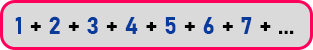The sum of first ‘7’ natural number is: 1 + 2 + 3 + 4 + 5 + 6 + 7 = 28. There is direct formula for that,Above is the direct formula to find the sum of first ‘n’ natural numbers but here we will not use that, we will find the sum by using for loop. So, we want to add the numbers one by one and find the sum. Before writing the program let us do some paperwork.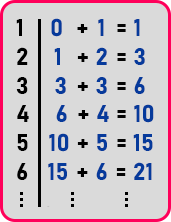1. first, we add ‘1’ with zero because there is no other number, so the result is ‘1’.
2. Now, add ‘2’ to the previous result that is ‘1 + 2 = 3’.
3. Now, again add ‘3’ to the result of the previous addition ‘3 + 3 = 6’.
4. Add ‘4’ to the previous result that is ‘6 + 4 = 10’.
5. Add ‘5’ to the result of the previous addition that is ’10 + 5 = 15’
6. Add ‘6’ to previous result that is ’15 + 6 = 21’

So, the sum of the first six natural numbers is 21. So, that’s how we can find the sum without using the formula. Now where to store the result of the addition? We will create a separate variable for this and keep modifying this again and again in each step. Let’s say the name of the variable is ‘sum’.

On the left-hand side, we are written numbers from 1 to 6. So, it is looking like a counter and these numbers are added to some another number in every step.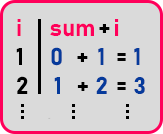##### Sum of N Natural Numbers Flowchart: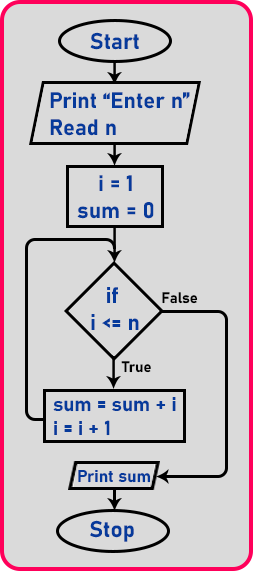So already we have written a flow chart here. Let us study that flow chart. First, we take a number from the user. Then we should initialize the counter to ‘1’ as well as our ‘sum’ variable to 0 which will be modified in every iteration of the loop. Then up to where we want to find the sum, let’s take that number be ‘n’. So, we will check the condition as ‘if (i <= n)’. If our counter is equal to the given number then the loop will be exit.

If the condition will true then modify the ‘sum’ variable as ‘sum = sum + i’ and increment the ‘i’ variable by 1. After this step control goes back to the condition and checks if the condition is true then again modify the ‘sum’ and ‘i’ variables and it will continue until the condition become false. If the condition will false, then simply stop the loop. Now let us look at the program.

##### Program to print sum of first N natural numbers using for loop in C++:
```#include <iostream>
using namespace std;
int main()
{
int n, sum = 0;
cout << "Enter number:" << endl;
cin >> n;
cout << endl;

for (int i = 1; i <= n; i++)
{
sum += i;
}
cout << "Sum N no. is " << sum << endl;
return 0;
}
```
###### Output: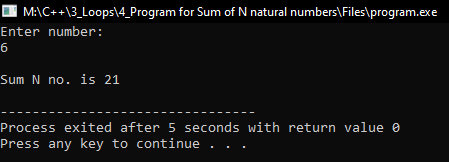##### Program to print sum of first N natural numbers using while loop in C++:
```#include <iostream>
using namespace std;
int main()
{
int n, i = 1, sum = 0;
cout << "Enter number:" << endl;
cin >> n;
cout << endl;
while (i <= n)
{
sum += i;
i++;
}
cout << "Sum N no. is " << sum << endl;
return 0;
}
```
###### Output: